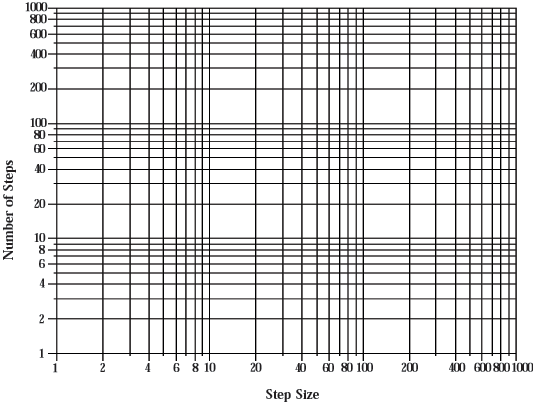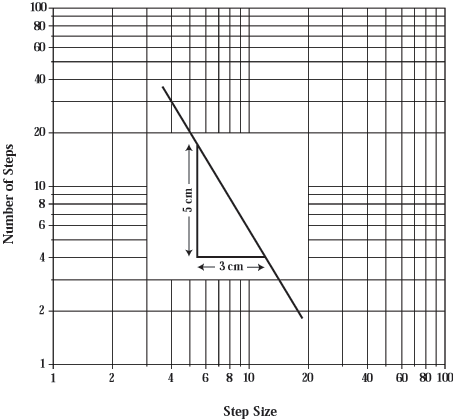HandsOn 2 - Measuring the Dimension of a Coastline

Now you are going to plot on graph paper the data you collected from your coastline measurements. A blank piece of graph paper for you to copy is given in Figure . This is a special kind of graph paper. If you have not used such graph paper before, the uneven scales may appear odd.Figure 2.2: Graph paper for plotting the entries in Table 2.1.

These scales, called logarithmic, are explained in the section Dimensions and Logarithms at the end of this unit. Basically, the numbers 2, 4, 8, 16, 32, 64,occur at equal intervals along each axis, as do the numbers 10, 100, 1000,When logarithmic scales are along both axes, the graph paper is called log-log paper. For now, you need to know only one thing about this novel scale: You can add a zero to all the numbers along either axis and the graph paper is equally useful. For example, the vertical axis can be relabeled 10, 20, 40, 100, 200, 400 1000, 2000, 4000, 10,000. The result is perfectly usable providing the numbers you are dealing with are in the corresponding range. Or you can multiply all numbers along either axis by 1/10. For example the vertical axis can be relabeled 0.1, 0.2, 0.4, 1, 2, 4, 10, 20, 40, 100. If the entries in your copy of the table do not match the numbers on one axis of the graph paper, just multiply all the numbers on that axis by 10 or by 1/10 until they do match.

1. Plot the numbers from your table on a copy of the graph paper. Plot the number of steps along the vertical axis and the step size along the horizontal axis. What kind of curve do the plotted points form? Do they lie approximately along a straight line? When they lie approximately along a straight line on this kind of graph, it is possible to define the object's dimension. The dimension is equal to the magnitude of the slope of this straight line. For some objects this slope-this dimension-is not a whole number. Objects with non-integer dimension are called fractals (meaning they have "fractional'' dimensions).

2. With a ruler, draw by eye the "best'' straight line using the points on your graph. Determine the magnitude of the slope of your graph. The slope is defined as
 slope = change in vertical rise along the line change in horizontal run along the line = "rise" "run" .
(2.1)
These vertical and horizontal changes are laid out using linear measures, not logarithmic. Use a regular ruler to measure this slope, not the scale along the axes as shown in the example of Figure 2.3.

 Q2.5: What is the magnitude of the slope (the dimension) of your coastline?Figure 2.3: Measurement of the slope of a straight line, using the linear coordinates of a regular ruler. The slope is negative, because for the positive "run'' there is a negative "rise'' (see Eq. 1).

 Q2.6: Why would a scientist care about the dimension of objects?

Now, to get a better feel for the process of formation of a coastline do at least one of the following two HandsOn activities. The first (HandsOn 3) is a group or classroom activity. The second (HandsOn 4) is an individual activity.

Next: HandsOn 3 - Creating a Rope Coastline